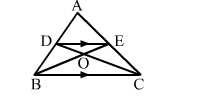# In the adjoining figure, DE || BC. Prove that

Question:

In the adjoining figure, DE || BC. Prove that
(i) ar(ACD) = ar(ABE),
(ii) ar(OCE) = ar(OBD),Solution:

DEC and ​∆DEB lies on the same base and between the same parallel lines.
So, ar(​∆DEC) = ar(∆DEB)                      ...(1)

(i) On adding​ ar(∆ADE)​ in both sides of equation (1), we get: